Publication date: 08/13/2020

# The Alias Matrix

The Alias Matrix entries represent the degree of bias imparted to model parameters by the effects that you specified in the Alias Terms outline. The Alias Matrix is also used in defining alias optimality. See Alias Optimality in the Custom Designs section.

Calculations for the Alias Matrix are based on the model matrix. See Model Matrix in the Custom Designs section.

Let X1 be the model matrix corresponding to the terms in the Model outline. Denote the matrix of model terms for the effects specified in the Alias Terms outline by X2.

The assumed model is given as follows: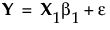Suppose that some of the alias terms are active and that the true model is given as follows: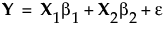The least squares estimator of β1 is given by: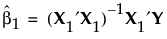Under the usual regression assumptions, the expected value of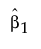is given by: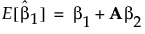where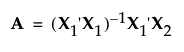.

The matrix A is called the alias matrix.